Courses

# Test: Determinacy And Indeterminacy -2

## 10 Questions MCQ Test Topicwise Question Bank for GATE Civil Engineering | Test: Determinacy And Indeterminacy -2

Description
This mock test of Test: Determinacy And Indeterminacy -2 for Civil Engineering (CE) helps you for every Civil Engineering (CE) entrance exam. This contains 10 Multiple Choice Questions for Civil Engineering (CE) Test: Determinacy And Indeterminacy -2 (mcq) to study with solutions a complete question bank. The solved questions answers in this Test: Determinacy And Indeterminacy -2 quiz give you a good mix of easy questions and tough questions. Civil Engineering (CE) students definitely take this Test: Determinacy And Indeterminacy -2 exercise for a better result in the exam. You can find other Test: Determinacy And Indeterminacy -2 extra questions, long questions & short questions for Civil Engineering (CE) on EduRev as well by searching above.
QUESTION: 1

### Match List-I (Structure) with List-ll (Degree of static indeterminacy) and select the correct answer using the codes given below the lists: List-I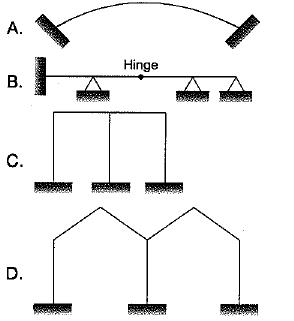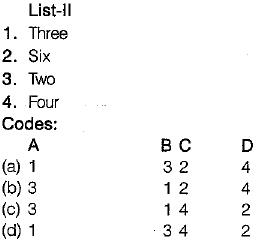Solution:

Correct List-II in the question!
List-II
Three
Six
Two
Five
Replace any one of the options with the option!
A   B   C   D
1    4   2    2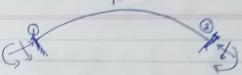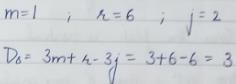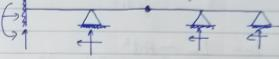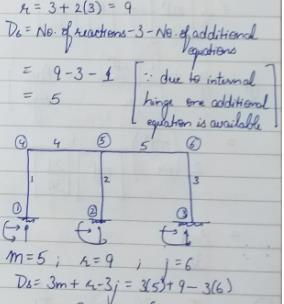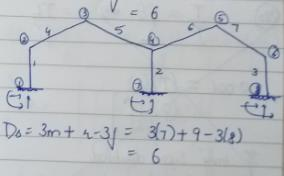QUESTION: 2

### A prismatic beam is shown in the figure given below.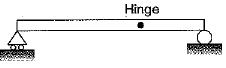​Consider the following statements: 1. The structure is unstable. 2. The bending moment is zero at supports and internal hinge. 3. It is a mechanism, 4. It is statically indeterminate. Which of these statements are correct?

Solution:

The degree of indeterminacy = 3 - 1 - 1 = -1 V So structure is deficient.and unstable. It is a mechanism,

QUESTION: 3

### The rigid-jointed frame shown in the figure is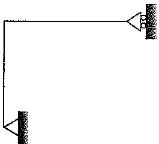Solution:
QUESTION: 4

What is the degree of static indeterminacy of the plane structure as shown in the figure below?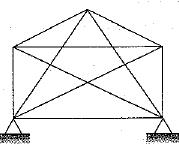Solution:

For plane truss degree of indeterminacy,
Ds = m + re - 2j
re = 4; m= 10; j = 5
Ds = 4 + 10 - 2 x 5 = 4

QUESTION: 5

Consider the following statements:
1. The displacement method is more useful when degree of kinematic indeterminacy is greater than the degree of static indeterminacy,
2. The displacement method is more useful when degree of kinematic indeterminacy is less than degree of static indeterminacy.
3. The force method is more useful when degree of static indeterminacy is greater than the degree of kinematic indeterminacy
4. The force method is more useful when degree of static indeterminacy is less than the degree of kinematic indeterminacy

Which of these statements is correct?

Solution:

Force method is useful when,
Ds < Dk
Displacement method is useful when,
Dk < Ds

QUESTION: 6

Which one of the following structures is statically determinate and stable?

Solution:

A structure will be statically determinate if the external reactions can be determined from force- equilibrium equations. A structure is stable when the whole or part of the structure is prevented from large displacements on account of loading.
The structure in figure (b) is stable but statically indeterminate to the second degree.
The structure shown in figure (c) has both reaction components coinciding with each other, so the moment equilibrium condition wilt never be satisfied and the structure will not be under equilibrium. In figure'(a), the structure is stable and there are three reaction components which can be determined by two force equilibrium conditions and one moment equilibrium condition.

QUESTION: 7

How is a truss, which undergoes rigid body translation for an arbitrary load, classified as?

Solution:
QUESTION: 8

The degree of static indeterminacy of the rigid frame having two internal hinges as shown in the figure below is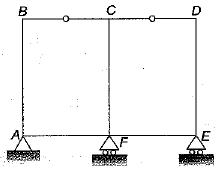Solution:

Ds=Dse+Dsi
Dss= rE- 3
= 4 - 3 = 1
Dsi = 3C - r= 3C - ∑(m1- 1)
= 3 x 2 -(2-1) +(2-1)
= 6 - 2 = 4
∴ Ds = Dss + Dsi = 5

QUESTION: 9

The pin-jointed frame shown in the figure is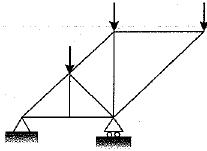Solution:

Degree of indeterminacy,
n = (m + rE) - 2j
= (9 + 3 ) - 2 x 6 = 0
Since the degree of indeterminacy is zero and the frame is stable so it is a perfect frame.

QUESTION: 10

What is the total degree of indeterminacy both internal and external of the plane frame shown below?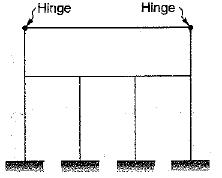Solution:

Ds = 3m + re- rr 3(j + j)
Number of members m=10
Number of external reactions re= 12
Number of interna! reaction components released
(rr) = 2
Number of rigid joints (j) = 8
Number of joints at which releases are located (j') = 2
∴ Ds = 3 x 10 + 12 - 2 - 3 x ( 8 + 2) = 10